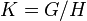# Quotient group need not be isomorphic to any subgroup

Jump to: navigation, search
This article gives the statement and possibly, proof, of a non-implication relation between two subgroup properties. That is, it states that every subgroup satisfying the first subgroup property (i.e., normal subgroup) need not satisfy the second subgroup property (i.e., endomorphic kernel)
View a complete list of subgroup property non-implications | View a complete list of subgroup property implications
Get more facts about normal subgroup|Get more facts about endomorphic kernel
EXPLORE EXAMPLES YOURSELF: View examples of subgroups satisfying property normal subgroup but not endomorphic kernel|View examples of subgroups satisfying property normal subgroup and endomorphic kernel

## Statement

It is possible to have a group$G$ (in fact, we can choose$G$ to be a finite group), and a normal subgroup$H$ of$G$ such that there is no subgroup of$G$ isomorphic to the quotient group$G/H$. In particular,$H$ need not be an endomorphism kernel in$G$.

## Proof

### Example of the quaternion group

Further information: quaternion group, subgroup structure of quaternion group, center of quaternion group

Suppose$G$ is the quaternion group$\{ 1,-1,i,-i,j,-j,k,-k \}$of order eight, and$H$ is the center of quaternion group$\{ 1, -1 \}$. Then$K = G/H$ is a Klein four-group. However, all the subgroups of order four in$G$ are isomorphic to cyclic group:Z4 and hence, in particular,$G$ has no subgroup isomorphic to$K$.